# ICSE BOARD PAPER-2016

To Access the full content, Please Purchase

• Q1

(a)  Fill in the blanks with the choices given in brackets.

(i)   Metals are good ___________(oxidizing/ agents/ reducing agents) because they are electron ________(acceptors/donors)

(ii)  Electrovalent compounds have _____ (high/low) melting points.

(iii) Higher the pH value of a solution, the more __________ (acidic/alkaline) it is.

(iv) ___________ (AgCl/PbCl2), a white precipitate is soluble in excess NH4OH.

(v)  Conversion of ethene to ethane is an example of _______ (hydration/hydrogenation)

(b)  Choose the correct answer from the options given below:

(i)   An element with the atomic number 19 will most likely combine chemically with the element whose atomic number is:

(a)  17                (b)  11

(c)  18                (d)  20

(ii)  The ratio between the number of molecules in 2g of hydrogen and 32g of oxygen is;

(a)  1:2               (b)  1:0.01

(c)  1:1               (d)  0.01:1

[Given that H =1, O=16]

(iii) The two main metals in Bronze are:

(a)  Copper and zinc       (b)  Copper and lead

(c)  Copper and nickel    (d)  Copper and tin

(iv) The particles present in strong electrolytes are:

(a)  only molecules         (b) mainly ions

(c)  ions and molecules  (d)  only atoms

(v)  The aim of the Fountain Experiment is to prove that:

(a)  HCl turns blue litmus red

(b)  HCl is denser than air

(c)  HCl is highly soluble in water

(d)  HCl fumes in moist air

(c)  Write balanced chemical equations for each of the following:

(i)   Action of warm water on AlN.

(ii)  Action of hot and concentrated Nitric acid on copper.

(iii) Action of Hydrochloric acid on Sodium bicarbonate.

(iv) Action of dilute Sulphuric acid on Sodium Sulphite.

(v)  Preparation of ethanol from Ethyl Chloride.

(i)   Dilute Hydrochloric acid is added to Lead nitrate solution and the mixture is heated.

(ii)  Barium chloride solution is mixed with Sodium Sulphate Solution.

(iii) Concentrated Sulphuric acid is added to Sugar Crystals.

(iv) Dilute Hydrochloric acid is added to Copper carbonate.

(v)  Dilute Hydrochloric acid is added to Sodium thiosulphate.

(e)  Identify the term/substance in each of the   following:

(i)   The tendency of an atom to attract electrons to itself when combined in a compound.

(ii)  The method used to separate ore from gangue by preferential wetting.

(iii) The catalyst used in the conversion of ethyne to ethane.

(iv) The type of reactions alkenes undergo.

(v)  The electrons present in the outermost shell of an atom.

(f)

(i)   A gas of mass 32 gms has a volume of 20 litres at S.T.P. Calculate the gram molecular weight of the gas.

(ii)  How much Calcium oxide is formed when 82 g of calcium nitrate is heated? Also find the volume of nitrogen dioxide evolved.

2Ca(NO3)2 2CaO + 4NO2 + O2

(Ca = 40, N = 14, O = 16)

(g)  Match the salts given in Column I with their method of preparation given in Column II:

 Column I Column II (i) Pb(NO3)2 from PbO (a) Simple displacement (ii) MgCl2 from Mg (b) Titration (iii) FeCl3 from Fe (c) Neutralisation (iv) NaNO3 from NaOH (d) Precipitation (v) ZnCO3 from ZnSO4 (e) Combination

(h)

(i)   Write the IUPAC names of each of the following:

1.

2.

3.

(ii)  Rewrite the following sentences by using the correct symbol > (greater than) or < (less than) in the blanks given:

1. The ionization potential of Potassium is ______ that of Sodium

2. The electronegativity of lodine is ______ that of Chlorine.

Marks:40

(a)

(i) Metals are good reducing agents because they are electron donors.

(ii)Electrovalent compounds have high melting points.

(iii)Higher the pH value of a solution, the more alkaline it is.

(iv)AgCl, a white precipitate is soluble in excess NH4OH.

(v)Conversion of ethene to ethane is an example of hydrogenation.

(b)

Explanation: The element with atomic number 19 has electronic configuration 2,8,8,1. Therefore, it is most likely to combine with element having atomic number 17 as its configuration will be 2,8,7 and it will have 7 electrons in its valence shell.

Explanation: Bronze is an alloy of copper and tin. It is used in making coins, sculptures etc.

Explanation:

2 g of hydrogen = 1 mole of hydrogen = 6.02 x 1023

32 g of oxygen = 1 mole of oxygen = 6.02 x 1023

Therefore, the ratio between the number of molecules in 2g of hydrogen and 32g of oxygen is 1:1

Explanation: The strong electrolytes dissociate completely. Therefore, solution of strong electrolytes has only ions.

Explanation: HCl is highly soluble in water. This property of HCl is displayed by fountain experiment with the help of water and red litmus solution.

(c)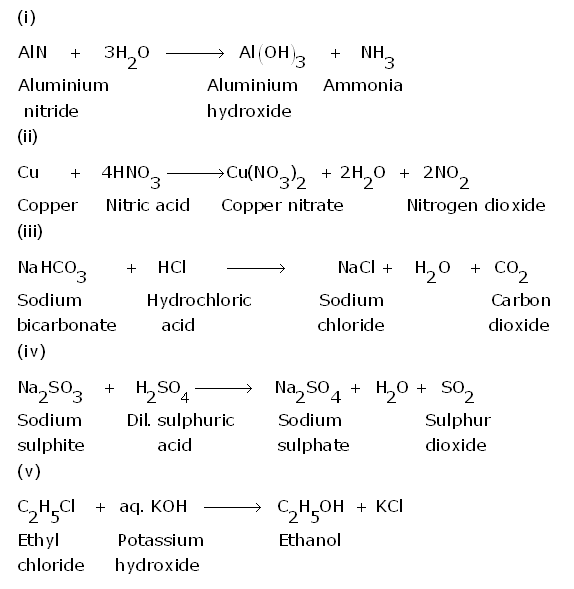(d)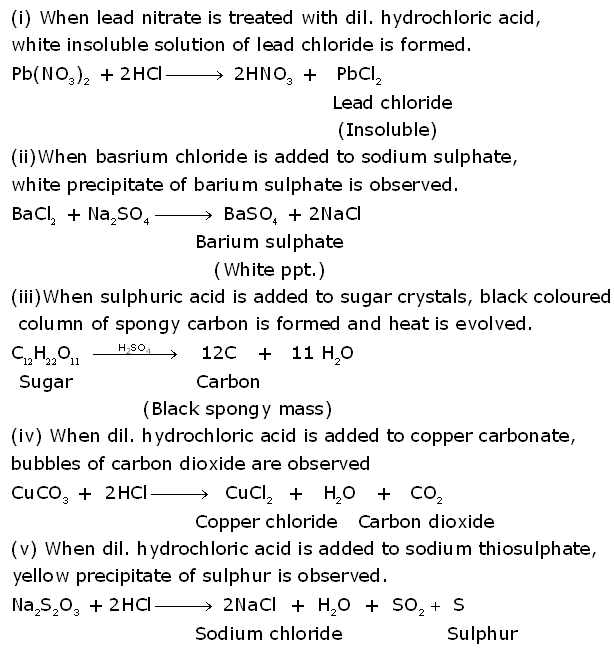(e)

(i) Electronegativity

(ii) Froth flotation method

(iii) Nickel

(v)Valence electrons

(f)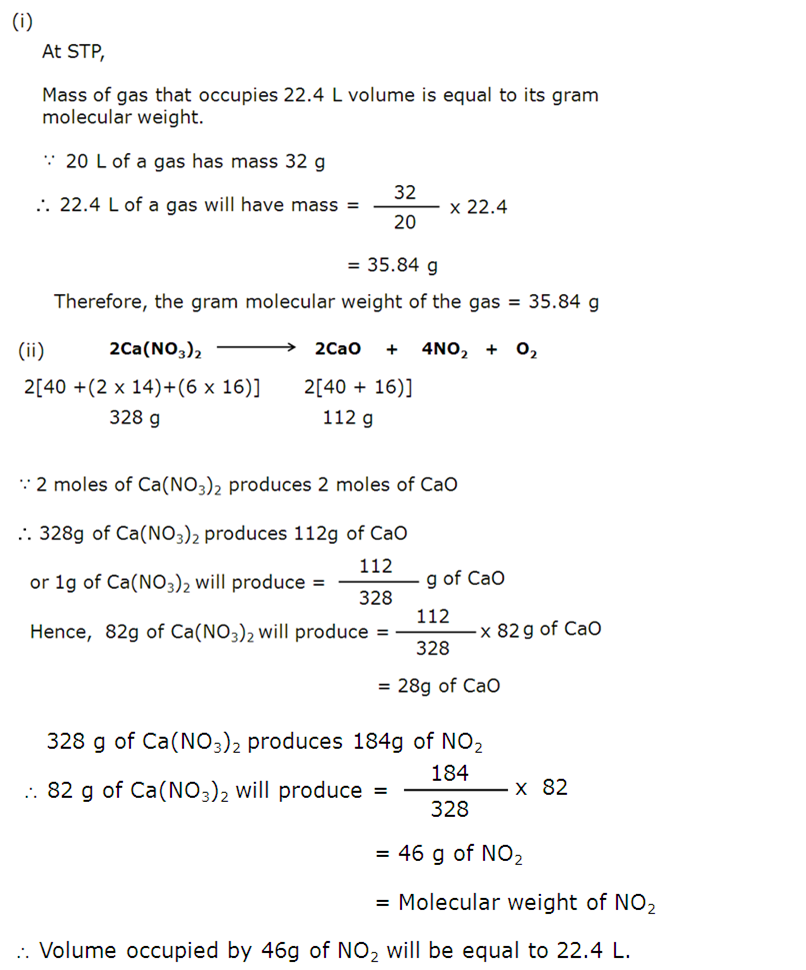(g)

 Column I Column II (i) Pb(NO3)2 from PbO (c) Neutralisation (ii) MgCl2 from Mg (a) Simple displacement (iii) FeCl3 from Fe (e) Combination (iv) NaNO3 from NaOH (b) Titration (v) ZnCO3 from ZnSO4 (a) Precipitation

(h)

1.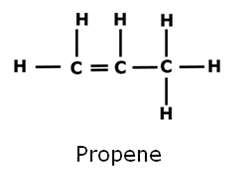2.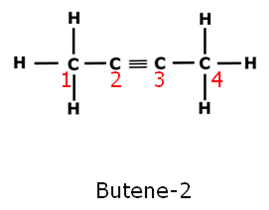3.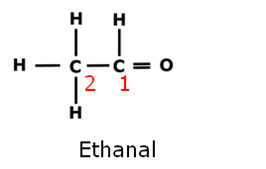(ii)

1. The ionization potential of Potassium is < (less than) that of Sodium.

2. The electronegativity of lodine is > (greater than)   that of Chlorine.

• Q2

(a)  Use the letters only written in the Periodic Table given below to answer the questions that follow:

(i)   State the number of valence electrons in atom J.

(ii)  Which element shown forms ions with a single negative charge?

(iii) Which metallic element is more reactive than R?

(iv) Which element has its electrons arranged in four shells?

(b)  Fill in the blanks by selecting the correct word from the brackets:

(i)   If an element has low ionization energy then it is likely to be __________ (metallic/non metallic)

(ii)  If an element has seven electrons in its outermost shell then it is likely to have the ________ (largest/smallest) atomic size among all the elements in the same period.

(c)  The following table shows the electronic configuration of the elements W, X, Y, Z:

 Element W X Y Z Electronic configurations 2,8,1 2,8,7 2,5 1

Answer the following questions based on the table above:

(i)   What type of Bond is formed between:

(1)  W and X                    (2)  Y and Z

(ii)  What is the formula of the compound formed between:

(1) X and Z               (2)  W and X

Marks:10

(a)

(i)5

(ii)M

(iii) T

(iv) T

(b)

(i) If an element has low ionization energy then it is likely to be metallic.

(ii) If an element has seven electrons in its outermost shell then it is likely to have the smallest atomic size among all the elements in the same period.

(c)

(i)

(1) Ionic

(2) Covalent

(ii)

(1)ZX

(2)WX

• Q3

(a)  Write a balanced chemical equation for each of the following:

(i)   Burning of ethane in plentiful supply of air.

(ii)  Action of water on Calcium carbide.

(iii) Heating of Ethanol at 170°C in the presence of conc. Suphuric acid.

(b)  Give the structural formulae of each of the following

(i)   2-methyl propane

(ii)  Ethanoic acid

(iii) Butan- 2-ol

(c)  Equation for the reaction when compound A is bubbled through bromine dissolved in carbon tetrachloride is as follows:(i)   Draw the structure of A.

(ii)  State your observation during this reaction.

(d)  Fill in the blanks using the appropriate words given below:

(Sulphur dioxide, Nitrogen dioxide, Nitric oxide, Sulphuric acid)

(i) Cold, dilute nitric acid reacts with copper to give __________.

(ii)  Hot, concentrated nitric acid reacts with sulphur to form ________

Marks:10

(a)(b)

(i)(ii)(iii)(c)

(i)(ii) During the reaction, reddish brown colour of bromine disappears.

(d)

(i) Cold, dilute nitric acid reacts with copper to give nitric oxide.

(ii) Hot, concentrated nitric acid reacts with sulphur to form sulphuric acid.

• Q4

(a)  Identify the gas evolved and give the chemical test in each of the following cases:

(i)   Dilute hydrochloric acid reacts with sodium sulphite.

(ii)  Dilute hydrochloric acid reacts with iron (II) sulphide.

(b)  State your observations when ammonium hydroxide solution is added drop by drop and then in excess to each of the following solutions:

(i)   copper sulphate solution

(ii)  zinc sulphate solution.

(c)  Write equations for the reactions taking place at the two electrodes (mentioning clearly the name of the electrode) during the electrolysis of:

(i)   Acidified copper sulphate solution with copper electrodes.

(ii)  Molten lead bromide with inert electrodes.

(d)

(i)   Name the product formed at the anode during the electrolysis of acidified water using platinum electrodes.

(ii)  Name the metallic ions that should be present in the electrolyte when an article made of copper is to be electroplated with silver.

Marks:10

(a)

(i) When dilute hydrochloric acid reacts with sodium sulphite, sulphur dioxide gas is evolved. It is a colourless gas having suffocating smell of burning sulphur. When the evolved sulphur dioxide gas is passed through potassium dichromate solution, the colour of the solution changes from orange to green. It confirms that the evolved gas is sulphur dioxide.

(ii) When dilute hydrochloric acid reacts with iron (II) sulphide, hydrogen sulphide gas evolves. It is a colourless gas with smell of rotten gas. When the evolved gas comes in contact with a paper dipped in lead acetate, the paper turns black. It confirms that the evolved gas is hydrogen sulphide.

(b)

(i) When ammonium hydroxide solution is added drop by drop to copper sulphate solution, pale blue precipitate of copper (II) hydroxide is formed.

But when ammonium hydroxide solution is added in excess, the pale blue ppt. of copper hydroxide dissolves and deep blue coloured solution of tetramminecopper (II)hydroxide is formed.

(ii)When ammonium hydroxide solution is added drop by drop to zinc sulphate solution, white precipitate of zinc hydroxide is formed.

But when ammonium hydroxide solution is added in excess, the white ppt. of zinc hydroxide dissolves and colourless solution of tetrammine zinc hydroxide is formed.

(c)

(i)

Electrolysis of acidified copper sulphate solution with

copper electrodes.

(ii) Electrolysis of molten lead bromide with inert electrodes.

(d)

(i) During the electrolysis of acidified water using platinum electrodes, oxygen gas is liberated at the anode.

(ii) Silver ions(Ag+)should be present in the electrolyte when an article made of copper is to be electroplated with silver.

• Q5

(a)  A gas cylinder contains 12 x 1024 molecules of oxygen gas.

If Avogadro's number is 6 x 1023; Calculate:

(i)   the mass of oxygen present in the cylinder.

(ii)  the volume of oxygen at S.T.P. present in the cylinder. [O = 16]

(b)  A gaseous hydrocarbon contains 82.76% of carbon. Given that its vapour density is 29, find its molecular formula. [C=12, H=1]

(c)  The equation 4NH3 + 5O2 ® 4NO + 6H2O, represents the catalytic oxidation of ammonia. If 100 cm3 of ammonia is used, calculate the volume of oxygen required to oxidise the ammonia completely.

(d)  By drawing an electron dot diagram show the formation of Ammonium Ion [Atomic No.: N = 7 and H = 1]

Marks:10

a.
(i)

(ii)

(b)

(c)

(d)

• Q6

(a)  Name the gas evolved when the following mixtures are heated:

(i)   Calcium hydroxide and Ammonium chloride

(ii)  Sodium nitrite and Ammonium chloride

(b)  Write balanced chemical equations for each of the following:

(i)   When excess of ammonium is treated with chlorine.

(ii)  An equation to illustrate the reducing nature of ammonia.

(c)  A, B, C and D summarize the properties of sulphuric acid depending on whether it is dilute or concentrated.

A = Typical acid property

B = Non volatile acid

C = Oxidizing agent

D = Dehydrating agent.

Choose the property (A, B, C and D) depending on which is relevant to each of the following:

(i)   Preparation of Hydrogen chloride gas.

(ii)  Preparation of Copper sulphate from copper oxide.

(iii) Action of conc. Sulphuric acid on Sulphur.

(d)  Give reasons why:

(i)   Sodium Chloride will conduct electricity only in fused or aqueous solution state.

(ii)  In the electroplating of an article with silver, the electrolyte sodium argento-cyanide solution is preferred over silver nitrate solution.

(iii) Although copper is a good conductor of electricity, it is a non-electrolyte.

Marks:10

(a)

(i) When mixture of calcium hydroxide and ammonium chloride is heated, ammonia (NH3) gas is liberated.

(ii) When mixture of sodium nitrite and ammonium chloride is heated, nitrogen (N2) gas is liberated.

(b)

(i)

(ii) In this reaction copper oxide is reduced to copper by ammonia.

(c)

(i) Preparation of Hydrogen chloride gas. B = Non volatile acid

(ii) Preparation of Copper sulphate from copper oxide. A= Typical acid property

(iii) Action of conc. Sulphuric acid on Sulphur. C = Oxidizing agent.

(d)

(i) Sodium Chloride will conduct electricity only in fused or aqueous solution state because in solid state its ions are held strongly and are not free to move.

(ii) In the electroplating of an article with silver, the electrolyte sodium argento-cyanide solution is preferred over silver nitrate solution because silver nitrate decomposes rapidly and the coating is not even.

(iii) Although copper is a good conductor of electricity, it is a non-electrolyte because when electric current is passed, it does not decompose and does not undergo any chemical change. Copper in molten state does not contain any free ions.

• Q7

(a)

(i)   Name the solution used to react with Bauxite as a first step in obtaining pure aluminum oxide, in the Baeyer's process.

(ii)  Write the equation for the reaction where the aluminum oxide for the electrolytic extraction of aluminum is obtained by heating aluminum hydroxide.

(iii) Name the compound added to pure alumina to lower the fusion temperature during the electrolytic reduction of alumina.

(iv) Write the equation for the reaction that occurs at the cathode during the extraction of aluminum by electrolysis.

(v) Explain why it is preferable to use a number of graphite electrodes as anode instead of a single electrode, during the above electrolysis.

(b) State what would you observe when:

(i)   Washing Soda Crystals are exposed to the atmosphere.

(ii)  The salt ferric chloride is exposed to the atmosphere.

(c)Identify the cations in each of the following case:

(i)   NaOH solution when added to the Solution (A) gives a reddish brown precipitate.

(ii)  NH4OH solution when added to the Solution (B) gives white ppt which does not dissolve in excess.

(iii) NaOH Solution when added to Solution(C) gives white ppt. which is insoluble in excess.

Marks:10

(a)

(i)Sodium hydroxide (NaOH) solution is reacted with Bauxite as a first step in obtaining pure aluminum oxide, in the Baeyer's process.

(ii)

(iii) Cryolite (Na3AlF6) is added to pure alumina to lower the fusion temperature during the electrolytic reduction of alumina.

(iv)

(v) During the electrolysis oxygen is evolved at anode. The graphite (carbon) anode is oxidised (to carbon dioxide) by oxygen evolved at anode. Therefore, it is preferable to use a number of graphite electrodes as anode instead of a single electrode, during the above electrolysis.

(b)

(i) When washing Soda Crystals (Na2CO3.10 H2O) are exposed to the atmosphere, they lose the water of crystalisation and form anhydrous salt (Na2CO3).

(ii) The salt ferric chloride is a deliquescent salt. When it is exposed to the atmosphere, it absorbs moisture (water).

(c)

(i)The cation present in solution (A) is ferric (Fe3+). The Fe3+ ions give brown ppt. of ferric hydroxide [Fe(OH)3] when NaOH solution is added to them.

(ii) The cation present in solution (B) is lead (Pb2+). The Pb2+ ions gives white ppt. of [Pb(OH)2]when NH4OH solution is added to them which does not dissolve in excess.

(iii) The cation present in solution (C) is calcium (Ca2+) The Ca2+ ions give white ppt. of calcium hydroxide [Ca(OH)2] when NaOH solution is added to them which is insoluble in excess .